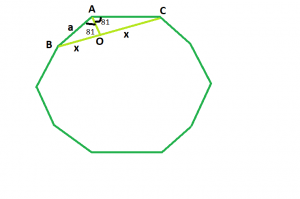# Diagonal of a Regular Decagon

Given an integer a which is the side of a regular decagon, the task is to find and print the length of its diagonal.Examples:

Input: a = 5
Output: 9.51

Input: a = 9
Output: 17.118

## Recommended: Please try your approach on {IDE} first, before moving on to the solution.

Approach: We know that the sum of interior angles of a polygon = (n – 2) * 180 where, n is the no. of sides in the polygon.
So, sum of interior angles of decagon = 8 * 180 = 1440 and each interior angle will be 144.
Now, we have to find BC = 2 * x. If we draw a perpendicular AO on BC, we will see that the perpendicular bisects BC in BO and OC, as triangles AOB and AOC are congruent to each other.
So, in triangle AOB, sin(72) = x / a i.e. x = 0.951 * a
Therefore, diagonal length will be 2 * x i.e. 1.902 * a.

Below is the implementation of the above approach:

## C++

 `// C++ program to find the diagonal ` `// of a regular decagon ` `#include ` `using` `namespace` `std; ` ` `  `// Function to return the diagonal ` `// of a regular decagon ` `float` `decdiagonal(``float` `a) ` `{ ` ` `  `    ``// Side cannot be negative ` `    ``if` `(a < 0) ` `        ``return` `-1; ` ` `  `    ``// Length of the diagonal ` `    ``float` `d = 1.902 * a; ` `    ``return` `d; ` `} ` ` `  `// Driver code ` `int` `main() ` `{ ` `    ``float` `a = 9; ` `    ``cout << decdiagonal(a) << endl; ` `    ``return` `0; ` `} `

## Java

 `// Java program to find the diagonal of a regular decdiagonal ` `import` `java.util.*;  ` `import` `java.lang.*;  ` `import` `java.io.*;  ` ` `  `public` `class` `GFG {  ` ` `  `    ``// Function to return the diagonal of a regular decdiagonal ` `    ``static` `double` `decdiagonal(``double` `a)  ` `    ``{  ` ` `  `//side cannot be negative ` `        ``if``(a<``0``) ` `        ``return` `-``1``; ` ` `  `        ``// length of the diagonal ` `        ``double` `d=``1.902``*a; ` `         `  `        ``return` `d;  ` `    ``}  ` ` `  `    ``// Driver code  ` `    ``public` `static` `void` `main(String[] args)  ` `    ``{  ` `        ``int` `a = ``9``;  ` `        ``System.out.println(decdiagonal(a));  ` `    ``}  ` `}  `

## Python3

 `# Python3 program to find the diagonal  ` `# of a regular decagon  ` ` `  `# Function to return the diagonal  ` `# of a regular decagon  ` `def` `decdiagonal(a) : ` ` `  `    ``# Side cannot be negative  ` `    ``if` `(a < ``0``) :  ` `        ``return` `-``1` ` `  `    ``# Length of the diagonal  ` `    ``d ``=` `1.902` `*` `a ` `    ``return` `d  ` ` `  `# Driver code  ` `if` `__name__ ``=``=` `"__main__"` `: ` ` `  `    ``a ``=` `9` `    ``print``(decdiagonal(a))  ` `     `  `# This code is contributed by Ryuga `

## C#

 `// C# program to find the diagonal of a regular decdiagonal ` `using` `System; ` ` `  `public` `class` `GFG {  ` ` `  `    ``// Function to return the diagonal of a regular decdiagonal ` `    ``static` `double` `decdiagonal(``double` `a)  ` `    ``{  ` ` `  `//side cannot be negative ` `        ``if``(a<0) ` `        ``return` `-1; ` ` `  `        ``// length of the diagonal ` `        ``double` `d=1.902*a; ` `         `  `        ``return` `d;  ` `    ``}  ` ` `  `    ``// Driver code  ` `    ``public` `static` `void` `Main()  ` `    ``{  ` `        ``int` `a = 9;  ` `        ``Console.WriteLine(decdiagonal(a));  ` `    ``}  ` `}  ` `// This code is contributed by anuj_67.. `

## PHP

 ` `

Output:

```17.118
```

My Personal Notes arrow_drop_upBudding Web DeveloperKeen learnerAverage CoderDancer&Social Activist

If you like GeeksforGeeks and would like to contribute, you can also write an article using contribute.geeksforgeeks.org or mail your article to contribute@geeksforgeeks.org. See your article appearing on the GeeksforGeeks main page and help other Geeks.

Please Improve this article if you find anything incorrect by clicking on the "Improve Article" button below.